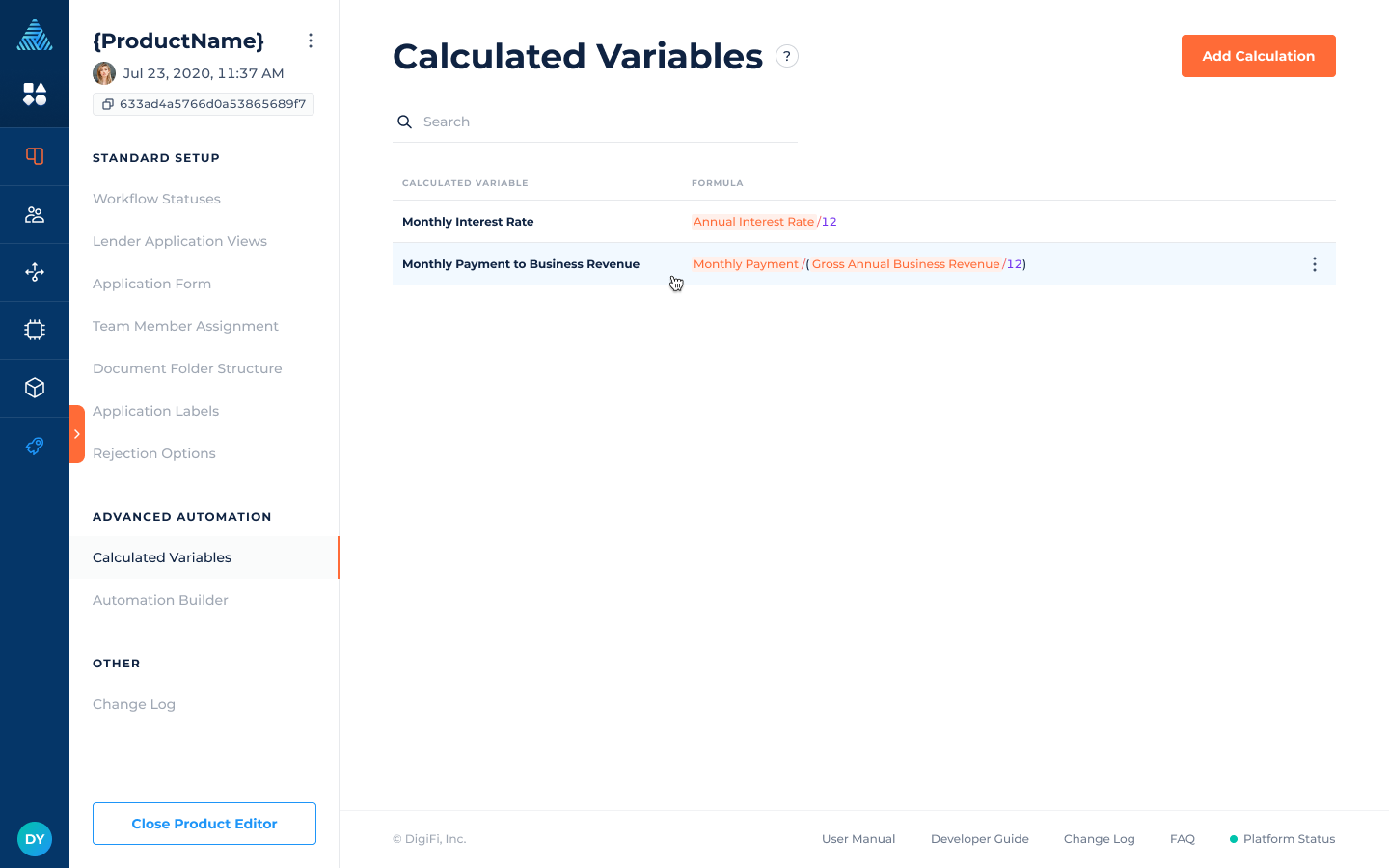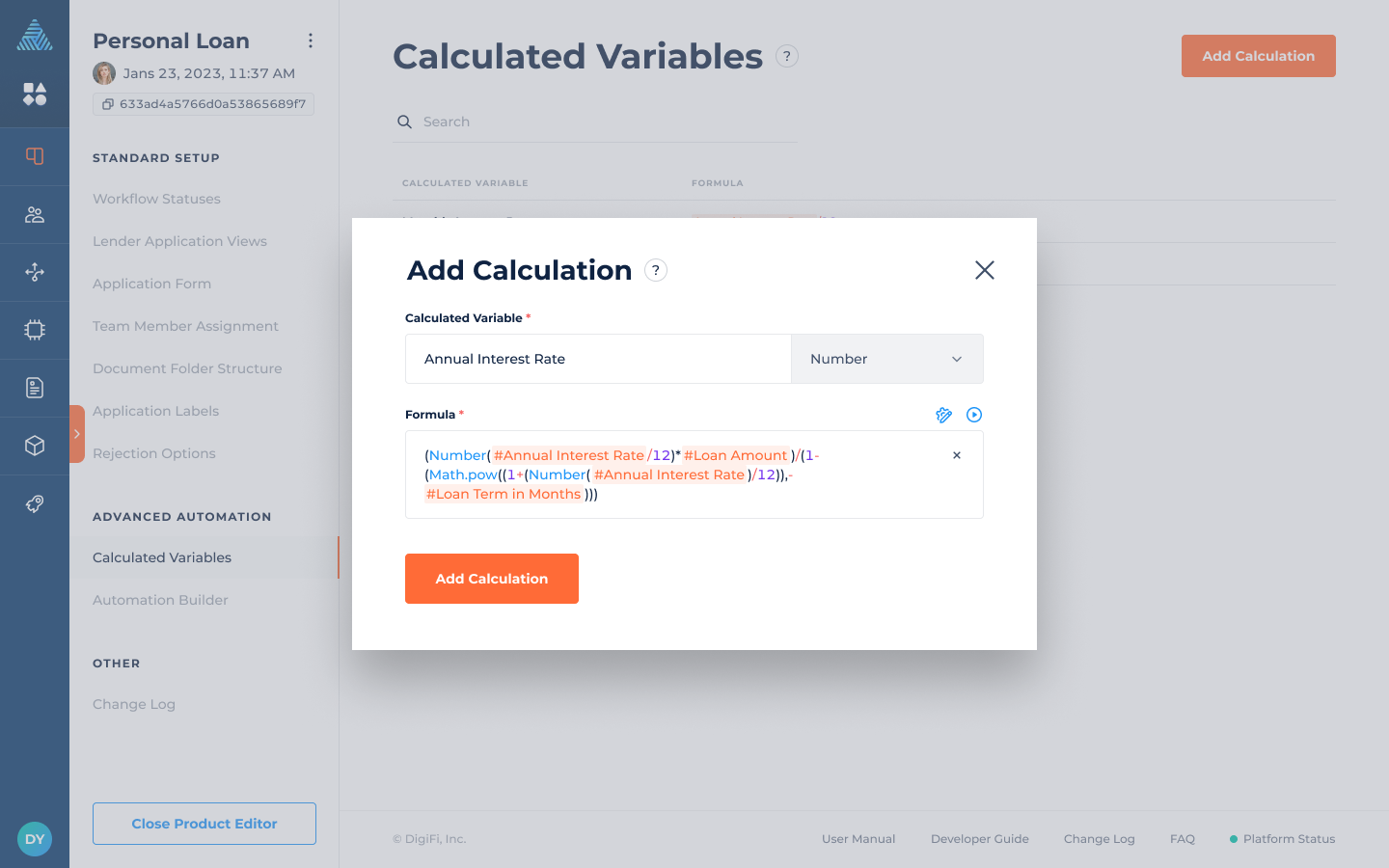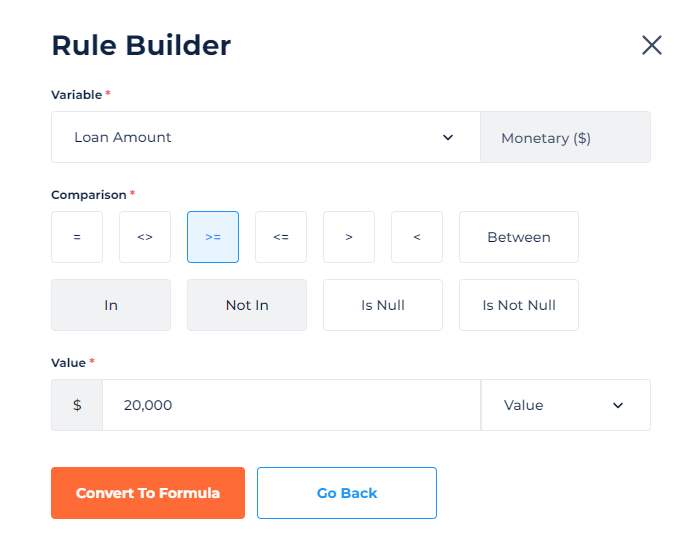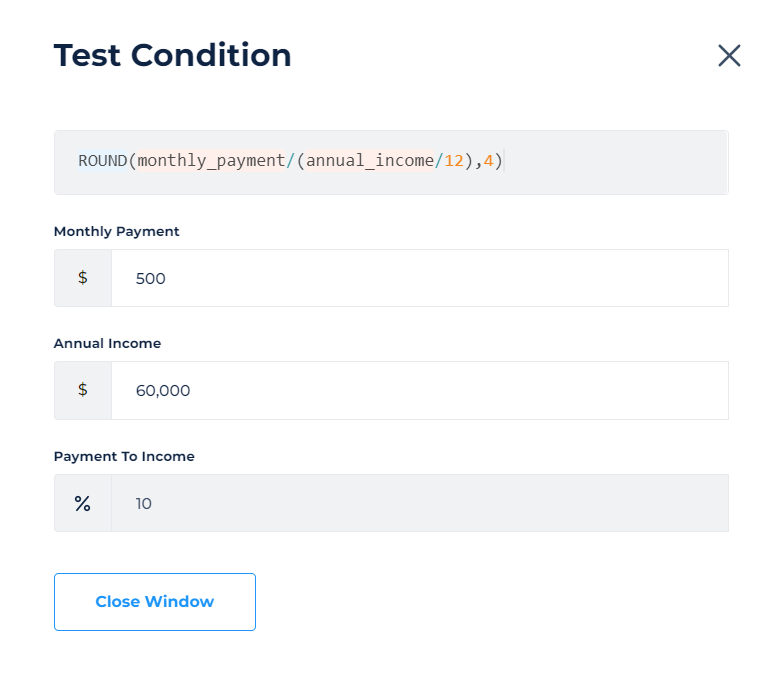# Calculated Variables

How to use calculated variables to automatically update application data.

## Overview of Calculated Variables

The "Calculated Variables" section of a loan products lets you use Excel-like formulas to automatically update variables. These formulas re-run every time application data is changed to keep your calculated data updated at all times.

If you have formulas that reference other formulas, the DigiFi platform automatically handles the chaining of logic. However, please note that you cannot add any circular logic.## How to Add a Calculation

To add a new calculated variable:

• Click the button.
• In the popup that appears, select a variable (where the result will be saved) and provide a formula using the DigiFi formula language.
• Click the button.By clicking the "gear" icon, you can use our rule-building tool to create a simple rule with a single comparative condition and convert the logic into a formula.By clicking the "play" icon, you can test your calculation to ensure your formula produces accurate results.If you are using table variable for calculations, to refer to a specific column cell in the table, use the following format:

``````table_variable_system_name[row_number].column_system_name
``````

Use the system names of the table variable or its columns. Remember that table row numbering starts from “0".

## How to Edit a Calculation

To edit a calculation:

• Click on the calculation in the table.
• Edit the calculation in the popup window.
• Click the "Save Changes" button.

Please note that changes to calculations will apply to future system actions but won't modify previously-run calculations within applications unless other edits are made to that application.

## How to Remove a Calculation

To remove a calculation:

• Hover over the calculation in the table.
• Click on the 3-dots icon on the right side.
• Select "Delete Calculation".